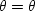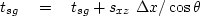Next: Units Up: Adjoint operators Previous: VELOCITY SPECTRA

# INTRODUCTION TO TOMOGRAPHY

Tomography is the reconstruction of a function from line integrals through the function. Tomography has become a routine part of medicine, and an experimental part of earth sciences. For illustration, a simple arrangement is well-to-well tomography. A sound source can be placed at any depth in one well and receivers placed at any depth in another well. At the sender well, we have sender depths s, and at the receiver well, we have receiver depths g. Our data is a table t(s,g) of traveltimes from s to g. The idea is to try to map the area between the wells. We divide the area between wells into cells in (x,z)-space. The map could be one of material velocities or one of absorptivity. The traveltime of a ray increases by adding the slownesses of cells traversed by the ray. Our model is a table s(x,z) of slownesses in the plane between wells. (Alternately, the logarithm of the amplitude of the ray is a summation of absorptivities of the cells traversed.) The pseudocode is


do s = range of sender locations
do g = range of receiver locations
z = z(s) # depth of sender.(s,g) 		 		 		# ray take-off angle.
do x = range from senders to receivers.# ray tracing
if modelingelse tomographyIn the pseudocode above, we assumed that the rays were straight lines. The problem remains one of linear operators even if the rays curve, making ray tracing more complicated. If the solution s(x,z) is used to modify the ray tracing then the problem becomes nonlinear, requiring the complexities of nonlinear optimization theory.Next: Units Up: Adjoint operators Previous: VELOCITY SPECTRA
Stanford Exploration Project
10/21/1998